# How to Count Cells that End With Specific Text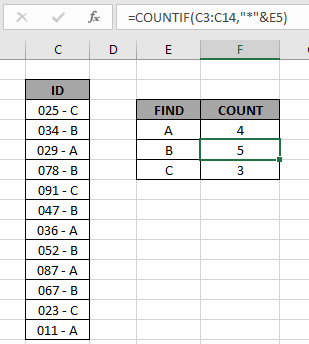In this article, we will learn how to count values which end with certain text in Excel. We will try to get the count of values that end with a given sub-string. This will require the use wildcards operators of Excel.

How to solve the problem ?

For this article we will be required to use the COUNTIF function. Here we are given some values in a range and specific text value as criteria. We need to count the values where the text in cell includes all the values which ends with the given text or pattern.

Generic formula:

= COUNTIF ( range, "*pattern" )

range: values given in as range

* : wildcards which find any number of characters within a given cell.

Pattern : given text or pattern to look for. Use patterns directly in the formula or else if you are using cell reference for the pattern use the other formula shown below.

Generic formula:

= COUNTIF ( range, "*" & pattern )

& : operator to concatenate the 2 values

Example:

All of these might be confusing to understand. So, let's test this formula via running it on the example shown below.

Here we have the ID records and we need to find the IDs which ends with A category.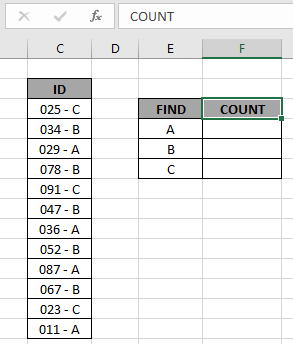Here to find the A category IDs, will be using the * (asterisk) wildcard in the formula. * (asterisk) wildcard finds any number of characters within lookup value.

Use the formula:

= COUNTIF ( C3:C14, "*A" )

OR

= COUNTIF ( C3:C14, "*" & E4 )

Explanation:

• COUNTIF function counts the cells given criteria
• Criteria is given  using * (asterisk operator) wildcard to look for value which has any number of characters before it.
• & operator concatenate the two values where it lays
• "*" & E4 : criteria, formula checks each cell and returns only the cells which satisfies the criteria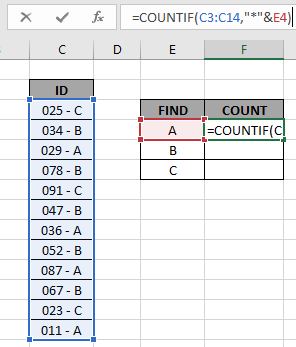Here the range is given as an array reference and pattern is given as cell reference. Press Enter to get the count.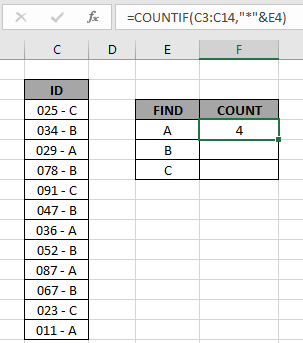As you can see the total values which ends with value A comes out to be 4.

Now copy the formula in other cells using the drag down option or using the shortcut key Ctrl + D as shown below.As you can see all the different category counts of IDs is there.

You can check which values end with a specific pattern in range using the excel filter option. Apply the filter to the ID header and click the arrow button which appears. Follow the steps as shown below.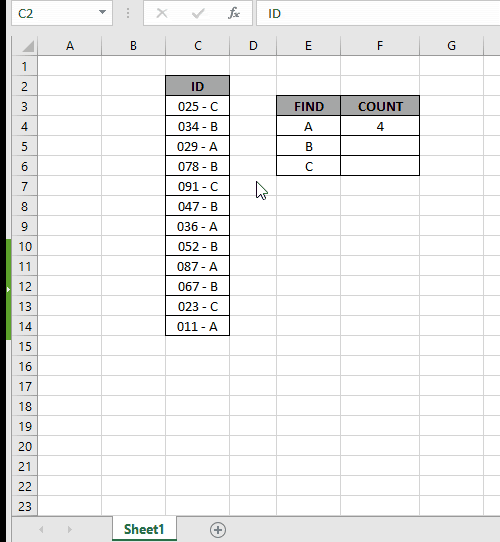Steps:

1. Select the ID header cell. Apply filter using shortcut Ctrl + Shift + L
2. Click the arrow which appeared as a filter option.
3. Deselect (Select All) option.
4. Now search in search box using the * (asterisk) wildcard.
1. Type *A in the search box and select the required values or select the (Select All search result) option as shown in the above gif.

As you can see in the above gif all the 4 values which match the given pattern. This also means the formula works fine to get the count of these values

Hope this article about how to Count values which end with using wildcards in Excel is explanatory. Find more articles on COUNTIFS functions here.

If you liked our blogs, share it with your friends on Facebook. And also you can follow us on Twitter and Facebook. We would love to hear from you, do let us know how we can improve, complement or innovate our work and make it better for you. Write us at info@exceltip.com

Related Articles

How to use the SUMPRODUCT function in Excel: Returns the SUM after multiplication of values in multiple arrays in excel.

COUNTIFS with Dynamic Criteria Range : Count cells dependent on other cell values in Excel.

COUNTIFS Two Criteria Match : Count cells matching two different criteria on list in excel.

COUNTIFS With OR For Multiple Criteria : Count cells having multiple criteria match using the OR function.

The COUNTIFS Function in Excel : Count cells dependent on other cell values.

How to Use Countif in VBA in Microsoft Excel : Count cells using Visual Basic for Applications code.

How to use wildcards in excel : Count cells matching phrases using the wildcards in excel

Popular Articles

50 Excel Shortcut to Increase Your Productivity

Edit a dropdown list

Absolute reference in Excel

If with conditional formatting

If with wildcards

Vlookup by date

Terms and Conditions of use

The applications/code on this site are distributed as is and without warranties or liability. In no event shall the owner of the copyrights, or the authors of the applications/code be liable for any loss of profit, any problems or any damage resulting from the use or evaluation of the applications/code.